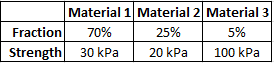## Composite Modulus

If a composite material has the known characteristics shown, and a strain of 0.10, what is its modulus of elasticity in kPa?Hint
$$\sigma_c=\sum f_i\sigma_i$$$where $$\sigma_c$$ is the strength parallel to the fiber direction, $$f_i$$ is the volume fraction of the individual material, and $$\sigma_i$$ is the individual material’s strength. Hint 2 Hooke’s Law: $$\sigma=E\varepsilon$$$
where $$\sigma$$ is the stress, $$E$$ is the elastic modulus (modulus of elasticity or Young’s modulus), and $$\varepsilon$$ is the strain.
A composite material is a material formed from two or more “base” materials. The base/basic materials have notably dissimilar physical or chemical properties, but when merged to create a composite, produce properties unlike the individual elements. To find a composite’s strength:
$$\sigma_c=\sum f_i\sigma_i$$$where $$\sigma_c$$ is the strength parallel to the fiber direction, $$f_i$$ is the volume fraction of the individual material, and $$\sigma_i$$ is the individual material’s strength. Thus, $$\sigma_c=f_1 \sigma_1+f_2\sigma_2+f_3\sigma_3$$$
$$=(0.7)(30kPa)+(0.25)(20kPa)+(0.05)(100kPa)$$$$$=21kPa+5kPa+5kPa=31\:kPa$$$
Hooke’s Law:
$$\sigma=E\varepsilon$$$where $$\sigma$$ is the stress, $$E$$ is the elastic modulus (modulus of elasticity or Young’s modulus), and $$\varepsilon$$ is the strain. Therefore, $$E=\frac{\sigma_c}{\varepsilon}=\frac{31kPa}{0.10}=310\:kPa$$$
310 kPa